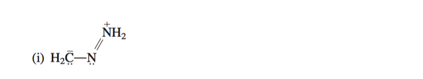Problem: Write a more stable contributing structure for each of the following. Use curved arrows to show how to transform the original Lewis formula to the new one. Be sure to specify formal charges, if any.

98% (69 ratings)
Problem Details

Write a more stable contributing structure for each of the following. Use curved arrows to show how to transform the original Lewis formula to the new one. Be sure to specify formal charges, if any.What scientific concept do you need to know in order to solve this problem?

Our tutors have indicated that to solve this problem you will need to apply the Major and Minor Resonance Contributors concept. If you need more Major and Minor Resonance Contributors practice, you can also practice Major and Minor Resonance Contributors practice problems .

What is the difficulty of this problem?

Our tutors rated the difficulty of Write a more stable contributing structure for each of the f... as low difficulty.

How long does this problem take to solve?

Our expert Organic tutor, Jonathan took 1 minute to solve this problem. You can follow their steps in the video explanation above.

What textbook is this problem found in?

Our data indicates that this problem or a close variation was asked in . You can also practice practice problems .